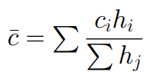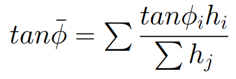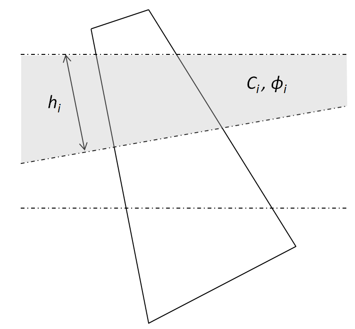The Rocscience International Conference 2021 Proceedings are now available. Read Now

# Sarma Interslice Strength

In both Sarma vertical slice and Sarma non-vertical slice methods, the factor of safety is affected by the strength parameters along the slice boundary. The values of cohesion and friction angle can be determined using Computed Average Value or User-Defined options.

## Computed Average Value

If the Computed Average Value option is selected, the cohesion and friction angle will be calculated automatically for each slice boundary.

The cohesion along the slice boundary is calculated using a weighted average:The friction angle along the slice boundary is estimated using a weighted average:where c_i and phi_i are the cohesion and friction angle of the material layer i along the slice boundary, and h_i is the length of the segment of the slice boundary that lies within that material layer. These variables are shown for one slice in the diagram below:## User-Defined

If the User-Defined option is selected, the cohesion and friction angle specified will be used instead of the average values for all slices.

## Limitations

If a slice boundary passes through an infinite strength material, the average strength parameters along the slice cannot be calculated using the Computed Average Values option. In this case it is recommended to use the User-Defined option for calculating the average strength parameters.